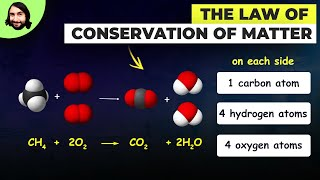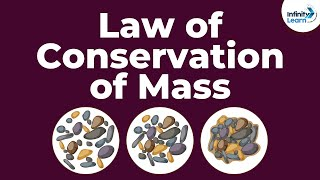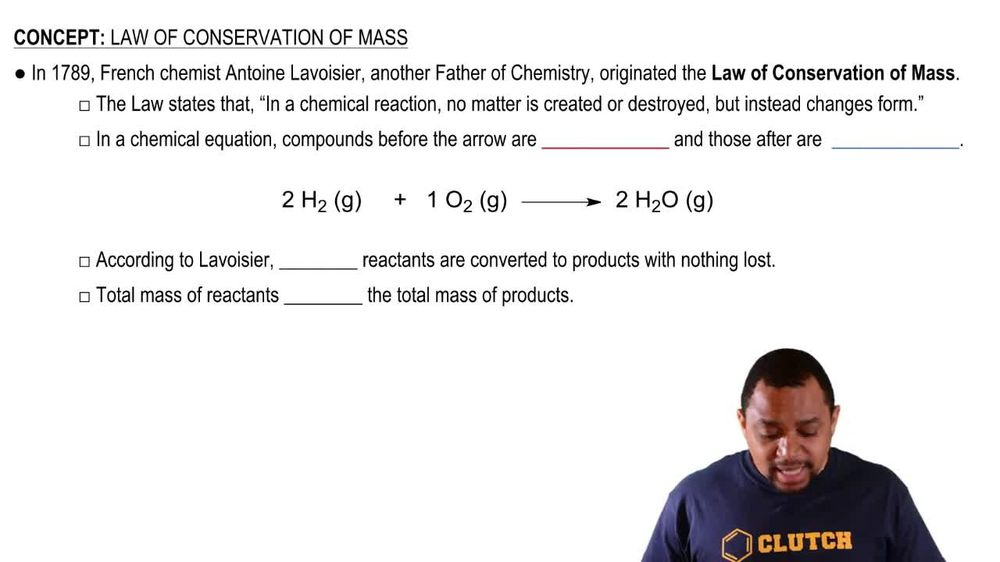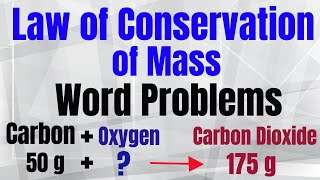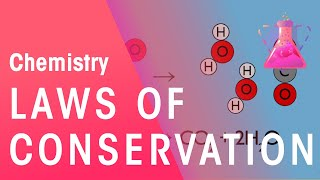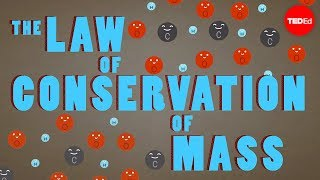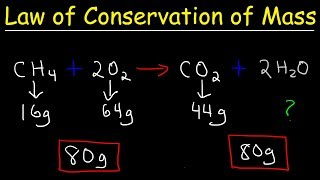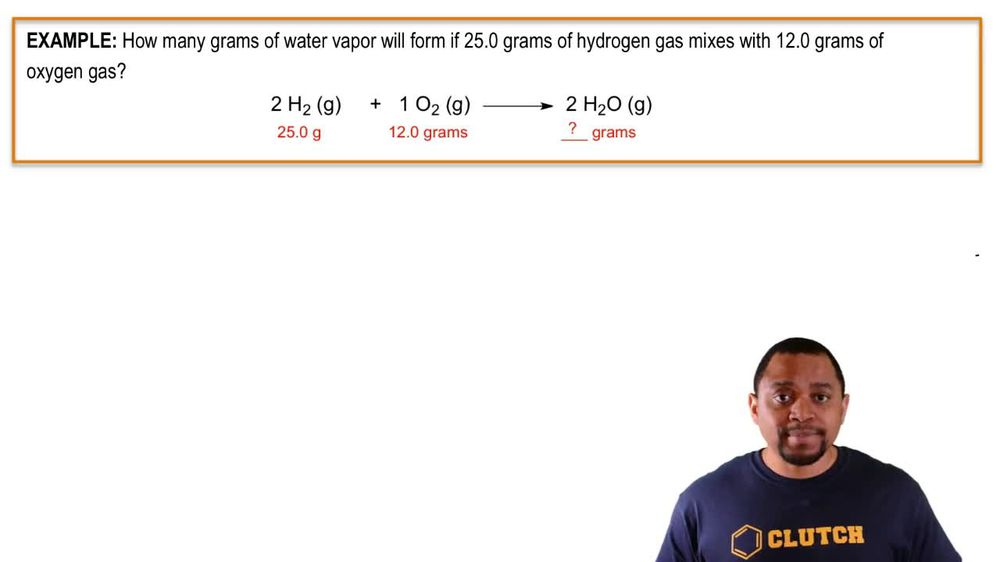Start typing, then use the up and down arrows to select an option from the list.
1. 2. Atoms & Elements2. Law of Conservation of Mass
Problem

# A sample of CaCO3 was heated, causing it to form CaO and CO2 gas. Solid CaO remained behind, while the CO2 escaped to the atmosphere. If the CaCO3 weighed 612 g and the CaO weighed 343 g, how many grams of CO2 were formed in the reaction?

Relevant Solution1m
Play a video:
Hi everyone. This problem tells us that a vessel is filled with 19.5 g of carbon monoxide. It was then allowed to completely react with 24g of oxygen, assuming that carbon dioxide is the only product that forms calculate its mass and grams So we have carbon dioxide as our product and we need to calculate its mass and we're told that we have to react ints 19.5 g of carbon monoxide and 24 g of oxygen. One thing to remember when we're dealing with this problem is the law of conservation of mass, which tells us that mass is conserved. So the sum of our reactant masses is the mass of our single product. So if we take the some 19. g of carbon monoxide and 24 g of oxygen, it is going to give us the mass of our single product carbon dioxide. So we have 43 0. g Of CO two. This is our final answer, and that is the end of this problem. I hope this was helpful.